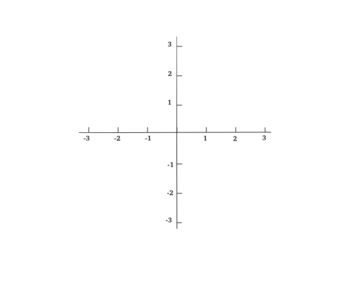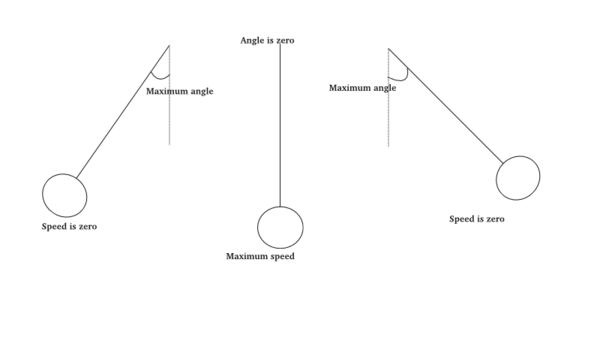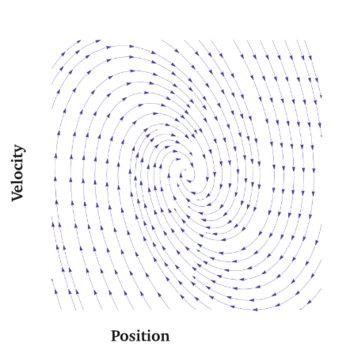# Coordinates: how symbols talk to geometry

by Rishidev Chaudhuri

Like the rest of us poor mortals, wandering in constant confusion between things and the names for things, bewitched by language and unable to resist it, mathematicians and physicists are constantly struggling with their representations and yet entirely reliant upon them to grasp the world.

Many of the fundamental intuitions that we start to describe the world with are geometric or spatial: this is a point; this is another point; walk in this direction to get from the first point to the second; this is the path a particle takes. If we want to make this precise, to describe and classify and manipulate and compute, we need to be able to make these statements precise. The simple act of drawing a pair of coordinate axes on a flat surface and using pairs of numbers to describe points is extraordinarily powerful, yoking algebra and symbolic manipulation to geometry and spatial intuition, and it unlocks for us a language within which to watch spatial and temporal processes unfold. Similarly, describing points on the surface of the Earth by pairs of numbers (latitude and longitude are the most common) allows us to specify locations relative to other locations, to calculate distances and trajectories and to describe and communicate quantities that vary across the surface of the Earth, like weather patterns and temperatures.But in picking a particular representation we've done a certain violence to the geometric structure we started with, by forcing an arbitrary layer of description on top. We might have decided to describe points relative to axes at right angles, like so:

But we could equally well have rotated the axes, or shifted the center, or chosen axes that were at other angles, like so:Similarly, the standard way to describe points on the surface of the Earth is by their distance from the equator (i.e. latitude) and their distance from a line perpendicular to the equator and passing through Greenwich (longitude), but I could choose to describe places by how far away they are from my house and in which direction relative to some local landmark. And this is how we generally give directions locally.

And so, now that we've introduced a way of describing space, we have to be careful that we don't get led astray by our representations, and that we keep separate the convenient descriptors that we use and the spatial and physical quantities that we're trying to describe. Depending on our system of representation, the particular coordinates attached to London and New York might vary dramatically. But our calculation of the physical distance between them shouldn't depend on how we've chosen to represent them.

Physicists and mathematicians have developed a lot of theory to derive and explain which quantities are physically meaningful (e.g. the distance between London and New York) and which quantities are simply consequences of the particular representation that we have chosen (e.g. the longitude of New York). This is often not trivial. For example, as Einstein famously found, the distance in space between events will be calculated differently by observers moving at different velocities (a form of coordinate dependence), but there is a quantity called the interval that combines the distance and time between events that all observers can agree on.

Once we have our coordinate systems and some notion of which quantities are physically meaningful and which aren't, we also have to figure out how to transform quantities from one coordinate system to another. This is both true of the accidental quantities, like the particular labels associated to points (which will depend on the particular coordinate system), and the essential ones, like the distance between points. The rules to calculate these essential quantities might depend on the particular coordinate system but the quantities that result from applying the rules shouldn't. So, for example, if we want to calculate the distance between two points, and our coordinate axes are perpendicular, we can just add the sums of the squares of the distances along each coordinate axis (this is Pythagoras' theorem). On the other hand, if our coordinate axes are at some other angle to each other, the distance calculation becomes more complicated (but some other computation might become simpler).

This is a general trope, and is a common strategy for attacking physical problems. Just like certain philosophical problems are intractable in the language of the Middle Ages and immediately transparent in modern language, the right choice of coordinate system can make a problem trivial to solve1.

Geometric ideas and spatial intuition are very valuable. Few people immediately grasp the meaning of a set of equations or symbols, and often when we say we understand something, we mean that we have a mental picture of it. We've used the introduction of symbolic representation to bring the computational machinery of algebra to geometry, but this step also opens up the possibility of going the other way, and attaching space and geometry to problems that are framed symbolically. The “spaces” that emerges in these situations are more abstract than physical space, but they carry with them many of the same structures — abstractions of geometric notions like distances, directions and trajectories. And many physical and mathematical problems share the same deep structure, one that is obscured by their particular representation but revealed by the abstract geometric structure underlying them (I will resist the temptation to draw some mystical neo-Platonic moral from this). The dual movement, from algebra and symbols to geometry and pictures and back is a fundamental pattern of mathematical thought and is almost always fruitful. If you've ever graphed a relationship (say the population of a country over time, or how the production of a country varies with its population), you've already taken advantage of the transformation from symbolic relationships into geometrical ones.

Studying the geometric structures associated with sets of equations that describe physical systems is particularly productive. As an example, consider the venerable favorite of the physicist: the pendulum. The structures underlying the behavior of a pendulum are ubiquitous2; roughly, they are the model for things that go round and round, or show repeated behavior.

So we start our pendulum off and let it swing. A convenient way to describe its position is by the angle it makes as it swings. Once we let the pendulum go, the angle will decrease from a maximum value to zero (in the center), then increase to the same maximum value on the other side and then return. When the angle is zero the pendulum will be going at its fastest, and when the angle is at its greatest the pendulum is stationary. This is a common pattern underlying oscillatory behavior. If this isn't intuitive, think of a swing –- the swing is fastest in the middle and hangs in the air at the ends before it changes direction. Similarly, the sun is moving north at its fastest during the vernal equinox and comes to a halt before changing directions during the solstice.So Let's say we plot these two things against each other: the position of the pendulum is on the x-axis and its speed is on the y-axis. If we take the idealized pendulum, which never stops, we'll find that they form a circle, moving from zero speed and maximum angle, to maximum speed and zero angle. For a more realistic pendulum, which eventually comes to a stop, we'll see it spiral into the center, eventually settling to zero speed and zero angle.

What can we do with this picture of the pendulum? More precisely, how can we use this space that we've described the pendulum's behavior in. Note that one direction in this space represents position and the other represents velocity3, so this space isn't a physical space. As a start, observe that we don't need more quantities to predict where the pendulum is going. Given its position and how fast and in what direction it's moving, we can predict where it's going to be next. So a two-dimensional abstract space is sufficient. This is what physicists mean when they talk about the dimension of a system.

How could we express our prediction of the system's behavior? Let's go back to our picture and at each point draw a little arrow describing the direction in which the system would move if it were to be that point, with the length corresponding to how fast it would move. Again, we're using geometric language, but we're describing the pendulum in a more abstract space. Each “point” is a combination of position and velocity and each direction is a mixture of changes in position and changes in velocity. Going “right” means changing position and not velocity, going “up” means changing velocity and not position and the intermediate directions are combinations of changes. The mathematical term for this set of arrows in this abstract space, which describes how the system will change, is a “differential equation”, and the way we'd usually solve this equation is by starting the system off at a point, following the arrow at that point a little bit to get to a new point and a new arrow, and repeating. Computers do this very well, once they are given coordinates to work with (and appropriate encouragement).So far we've taken a physical system and, by plotting some of its aspects against each other, represented it in some abstract space. What if we take this space seriously, as a geometric object in its own right? The aspects of the system that we've used to enter into this space are coordinates (here, position and velocity), describing points in the space, and we can start to ask the same sorts of questions as we did before. Are these good coordinates? Are there other representations that would serve our purposes better? Are there fundamental relationships in this space that must be preserved, relationships between points or along the paths the system takes? As one might imagine, these are all very useful questions. For example, as with our calculations on physical space, looking for good coordinates is often the way to solve differential equations: with certain sets of coordinates the behavior of the system looks very simple and its paths can be described very easily.

Once you start to look for them, abstract spaces are everywhere. For a very different example, consider a set of pictures of faces. Say we're taking low resolution pictures of faces with a 100×100 pixel camera. Each picture consists of a set of 10,000 numbers, with each number corresponding to the color at a particular point4 . The space of face pictures can be thought of as a geometric space, too, with 10,000 coordinates corresponding to the 10,000 points in each picture. Rather than the two-dimensional space that the pictures are representing, we now have a 10,000 dimensional space and each picture is a single point in this space. And, again, we can start to ask geometric questions. For example, is there a meaningful notion of distance in this space, perhaps based on how similar two pictures are. We can also start to interrogate our coordinates. For example, if I was describing a face (or a picture of a face) to you, I'd be unlikely to give you a list of color values at each point. Instead, I might give you a “value” for hair color, another one for nose shape and a third for hair style. This is another coordinate system. In theory, if I wanted to describe the face completely, I'd need to give you many more coordinates; for example, these few coordinates don't tell you whether the face has freckles and what the position of particular freckles are. But here we've packed most of the information into the first few coordinates (nose-shape, eye-color); this is unlike in the list-of-pixels set of coordinates, where any given subset of coordinates (values of pixel color in the middle of the face, for example), is relatively uninformative. This is another possible criterion for what makes a coordinate system valuable; if there's some underlying structure in the space, then capturing as much of that structure as possible in the first few coordinates is often a good way to proceed, especially if we don't care about exactly representing points in the space (here, faces) but rather, just want to represent them approximately and efficiently for some particular task (for example, I don't need to know the exact pattern of freckles on someone's face to identify them). And there exist procedures going the other way: given a set of points in some space, represented by some coordinate system, how do we find a new coordinate system that efficiently captures the location of a point using only a few coordinates5.

A number of people tell me they loved geometry in school, and seem puzzled and somewhat sad that their later mathematical education seems to have degenerated into a series of symbolic manipulations and computations. To a large extent, this is a consequence of the particular topics chosen for high school mathematics and of the fact that many math classes are taken as preparation for calculations in introductory physics or economics. But geometry doesn't disappear, and the grand currents of geometric thought multiply and spread out as one goes further, running through much of modern mathematics. One of the most exhilarating intellectual moments is when a profusion of symbols and equations suddenly resolves itself into a simple moment of underlying geometric intuition, a picture that seems both profound and inevitable. And for whatever reason, the intuition of space, seemingly one of the most primitive and concrete intuitions we have, reveals itself hidden behind our most abstract thoughts.

————————————————

1How this happens, and which coordinate systems should correspond to which problems is a fascinating subject, and one that involves its own mathematical machinery. As an example, many problems that involve multiple interacting components in one coordinate system separate into a series of simpler non-interacting subproblems in the right coordinate system. It is hard to exaggerate how useful this idea has been.

2I should note that I'm talking about an idealized pendulum that has been displaced only a small amount from its center. See the Wikipedia page on “harmonic oscillator” if you're curious. At times it can seem like most of an undergraduate physics education is encountering harmonic oscillators in increasingly abstract contexts.

3I'm being sloppy with units.

4Or, if this picture is in color, each of the 10,000 points has three numbers. The three numbers are often the values of red, green and blue at that point. But note that this is a particular set of coordinates used to describe the (abstract) space of colors, and there exist other coordinate systems, adapted for different purposes, and formulas for moving between them. See http://en.wikipedia.org/wiki/Color_space , for example.

5For a fun illustration of this using faces, see http://en.wikipedia.org/wiki/Eigenface